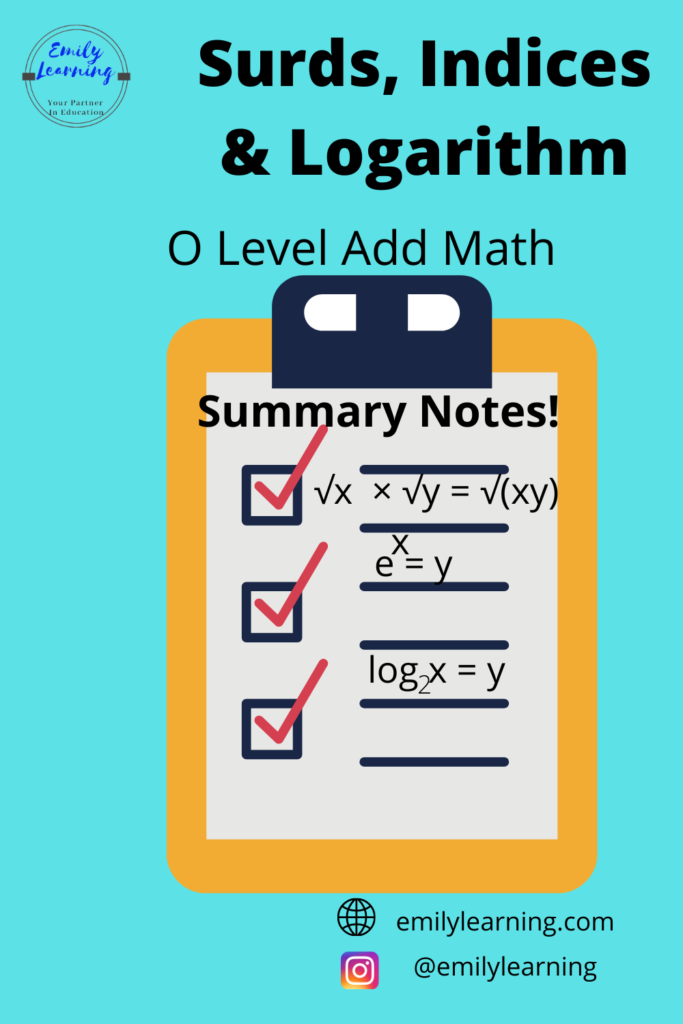# Summary Notes for Surds, Indices and Logarithm (O Level Add Math)Here, you will find the summary notes for Surds, Indices, and Logarithm written based on O Level Additional Mathematics Syllabus.

We talked about what’s in the syllabus for these topics in the article here. Most of what’s covered in these topics are also covered in the Mathematics syllabus for O Level, so do revise, or at least memorize the formulae in Mathematics when you are revising O Level Additional Mathematics.

In my course on Additional Mathematics I’ll go through all the essential formulae that you’ll need to know for O Level Add Maths (including those that are already covered in elementary mathematics). You can get my course here.

For students without Add Math background, and who are taking H2 Mathematics, surds, indices, and logarithms are assumed knowledge and can be combined with any topic. Similarly, for students who are going to take H2 Mathematics in A Levels, this is an important topic.

Let’s look at the concepts you need to know for surds, indices, and logarithm. Do note what you have learned in indices for elementary math is assumed knowledge for this topic.

## Surds for O Level Additional Mathematics

In O level Additional Mathematics, we will be looking at surds involving square roots.

### Addition and subtraction of surds:

We can only or subtract surds if what’s inside the surd sign is completely the same.a√x + b√x = (a+b)√xa√x -b√x = (a-b)√x

√x  × √y = √(xy)

### Rationalize denominators with surds

If the denominator is a ± b√x then you will rationalize it with a∓ b√x

## Indices for O Level Additional Mathematics

m ×  a n = a m+n

m ÷ a n = a m-n

### Multiplication for indices with same power:

m ×  b m = (ab) m

### Division for indices with same power:

m ÷ b m = (a/b) m

### When solving equations involving indices,

If a m = a n then m = n

## Rules of Logarithm for O Level Additional Mathematics

m = y <==> logay = m
logax +logay = loga(xy)logax – logay = loga(x/y)rlogax = logaxr
Change of baselogax  = (logbx )/(logba )

• Natural logarithm is ln (base e).
• Common logarith is log (base 10).

### When solving equations involving logarithm:

if logax = logay   <==> x = y

## Want to learn O Level Additional Math on demand?

Knowing the concepts and formulae above is not enough for you to even pass the O Level Additional Mathematics exams. You need to know how to apply these concepts. So, you’ll definitely need to try questions.

In my O Level Additional Math course, I go through the concepts, how to apply them, and what concept to use for each type of question. With these skills, students finish the course as confident students, able to apply what they have learned to tests and exams. Check out the course here.